##### Basic Math & Pre-Algebra All-in-One For Dummies (+ Chapter Quizzes Online)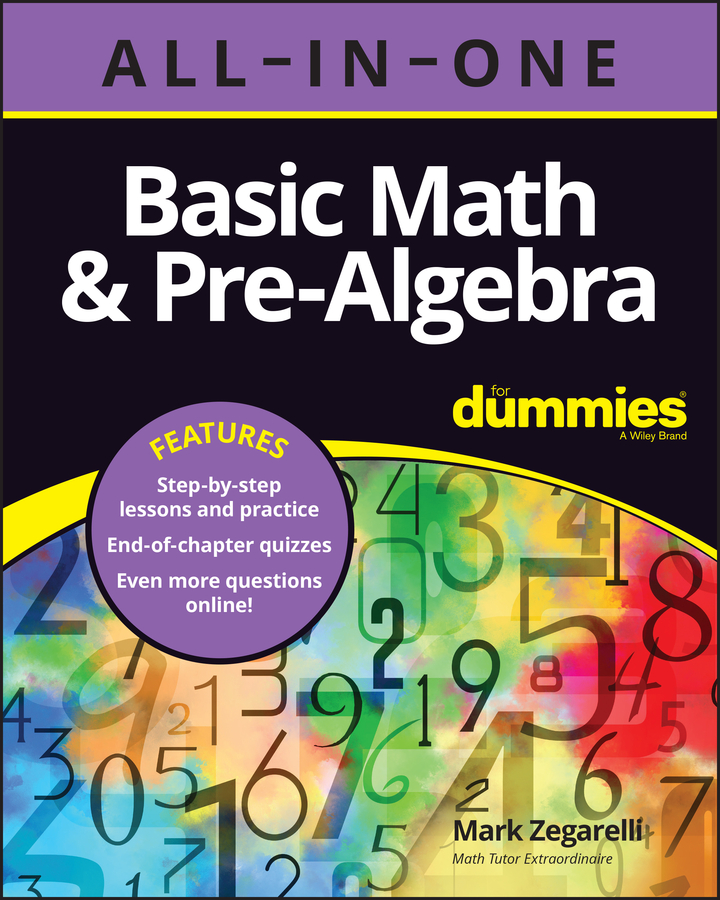Cross-multiplication is a handy math skill to know. You can use it for a few different purposes. For example, you can compare fractions and find out which is greater.

To cross-multiply two fractions:

1. Multiply the numerator of the first fraction by the denominator of the second fraction and jot down the answer.

2. Multiply the numerator of the second fraction by the denominator of the first fraction and jot down the answer.

For example, suppose you have these two fractions: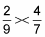When you cross-multiply, you get these two numbers: 2 7 = 14 and 4 9 = 36

You can use cross-multiplication to compare fractions and find out which is greater. When you do so, make sure that you start with the numerator of the first fraction.

To find out which of two fractions is larger, cross-multiply and place the two numbers you get, in order, under the two fractions. The larger number is always under the larger fraction.

For example, suppose you want to find out which of the following three fractions is the greatest: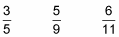Cross-multiplication works only with two fractions at a time, so pick the first two: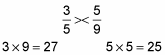Because 27 is greater than 25, you know now that 3/5 is greater than 5/9. So you can throw out 5/9.

Now do the same thing for 3/5 and 6/11: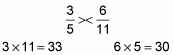Because 33 is greater than 30, 3/5 is greater than 6/11. Pretty straightforward, right?